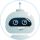# Mohr’s Circle Solution for the Strain Gauge Rosette

• Pages 3
• Words 607
• Views 417
• Pages 3
• Words 607
• Views 417

Get original paper in 3 hours and nail the task

124 experts online

## Quick and Dirty

Mohr’s Circle Solution for the Strain Gauge Rosette A 3 gauge rosette is attached to a simple tension bar. The three gauges of the rosette are at 45 degrees in relation to each other but the rosette is not aligned with the strap. The strap is 1. 00 inches wide and 0. 25 inches thick and is loaded with 3000 lbs tension with the force aligned with the long axis of the bar. The material is steel with: E = 29E6 psi and ? = 0. 3

## Theory

The theory is that the stress in the bar is uniaxial with the principal stresses being equal to P/A and zero.

The strains are biaxial with the maximum being P/AE and the minimum being – ? P/AE. The first principal stress and strain will be aligned with the force and the long axis of the bar. ?1 = P/A = 3000/(1. 00×0. 25) = 12,000 psi ? 2 = 0 psi ? 1 = P/AE = ? 1/E = 12,000/29E6 = 0. 000414 in/in = 414 µ? ?2 = – ? P/AE = – ? ?1/E = – ? ?1 = -0. 3×414 = 124 µ? Test Data: When loaded with 3000 lbs tension the three strain readings were: ? A = 310 µ? ?B = -20 µ? ?C = 26 µ? 10/27/2008

## Solution

Gauges A and C are perpendicular and since angles are doubled on Mohr’s circle, they will plot 180 degrees apart on the circle and define a diameter. A and C form a diameter of the circle, the center of the circle will be halfway between them. Center at (310 + 26)/2 = 168 µ? Find the offset from the center to gauges A and B 310 – 168 = 142 µ? -20 – 168 = -188 µ?

These can be used to find the radius Radius = [ 1422 + (-188)2 ]1/2 = 235 µ? Now we can find the principal strains ? 1 = center + radius = 168 + 235 = 404 µ? ?2 = center – radius = 168 – 235 = -67 µ?

Using the center and the radius, draw Mohr’s circle and draw three lines to represent the gauge readings. Consider a diameter of the circle connecting A and C. This diameter will have a perpendicular extending to B. There is only one way that this can be arraigned such that the counter-clockwise sense, ABC, of the rosette is preserved. In our case, the diameter connecting A and B must slope upward to the right (not downward to the right). We can now locate the exact three points on the circle that represent the directions of A, B, and C.

Remember that a point on the circle quantifies the normal and shear strains associated with a particular direction. Any location on the circle represents the same point on the structure and the same condition of strain or stress – just a different orientation for the coordinate system used to quantify the strains or stresses. Note that the shear coordinates of points A, B, and C are the offsets determined above and used to calculate the radius. This is because the vertical and horizontal projections of the 3 radii associated with points A, B, and C form equal triangles.

The completed Mohr’s circle of strain looks like this: Mohr’s Circle of strain -300 -200 20-100 310 Now we can calculate the principal stresses from the biaxial form of Hooke’s law.

?x = E (? x + v ? y ) 1? v2 E ? y = (? y + v ? x ) 1? v2 -100 68- 0 0 100 200 168 300 400 404 500 And 100 200 26 ?1 = 12,100 psi ? 2 = 2100 psi

We have some error in the magnitude of principal stresses. The direction of the first 300 principal stress can be found from Mohr’s circle of strain above. The angle from gauge A (@ +310 µ? ) to ? is clockwise and the cosine of the angle is:

Horizontal component/radius = (310 – 168) / 235 = 0. 604 Since the angle on the circle is double the physical angle, we want ? the angle to locate the direction of the first principal stress ? 1 in reference to gauge A. ?P =? arcos (0. 604) = 26. 4o

Our solution shows the first principal stress 0. 4 degrees clockwise from the theory. The first principal stress is 100 psi in magnitude off from the theoretical value and the second is 2100 psi off.

This essay was written by a fellow student. You may use it as a guide or sample for writing your own paper, but remember to cite it correctly. Don’t submit it as your own as it will be considered plagiarism.

## Need a custom essay sample written specially to meet your requirements?

Choose skilled expert on your subject and get original paper with free plagiarism report

Without paying upfront

### Related Topics